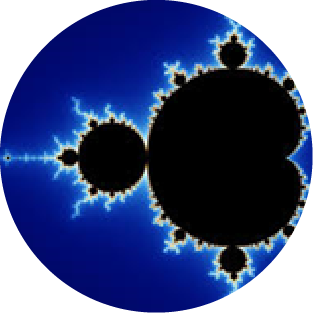## Quantum ComputingIn 1959, the late Richard Feynman in his lecture “There’s Plenty of Room at the Bottom” stated the possibility of using quantum effects for computation, and ever since, many breakthroughs in quantum computing have been made.  Often cited is the work of Steve Shor, whose “Shor Algorithm” realizes in theory the ability of a quantum computer to factor very large numbers in a very short order of time thereby possibly defeating encryption standards in widespread use.

Since these early achievements, many labs have demonstrated limited success, for example using Shor’s algorithm to factor numbers as large as 143, but clearly it is early in the development of the full potential of quantum computing.  But quantum computing is also poorly understood, and rightly so; it requires an advanced degree in physics to understand many of the principles being applied, and even then, it can be quite mysterious and unexplainable!

But one often overlooked fact is that quantum computers do not rely on digital arithmetic, instead, they perform computation using “quantum states and principles”.  For example, the collapsing energy states of qubits is not at all the manipulation of a digital number system; at present, it is better to describe collapsing energy states of qubits as resulting in a digital number representation.  Many types of quantum computing are probabilistic, whereas a superposition of qubit states collapse into a classical binary representation when a measurement of the quantum system is made.  Typically, a binary computer is then used to check or verify the result of quantum computation as it may not be correct each time the algorithm is run.  By configuring a quantum system appropriately, different types of problems can be represented and solved using quantum states and quantum computation principles.

Unfortunately, because of the probabilistic nature of quantum computing and many other present-day obstacles, the ability to operate on digital numbers and perform digital arithmetic is very limited on present-day quantum computers.  To be clear, classical computers excel in performing digital arithmetic while quantum computers do not.  Some progress is being made on quantum gates, and in the future, such developments may lead to hybrid quantum/classical computers which can solve some parts of digital arithmetic using quantum arithmetic.  It should be noted that some early progress has been made using modular arithmetic within the framework of quantum computation.

But most quantum researchers have been steadfast in finding quantum states that are binary in nature.  In fact, a “qubit”, while capable of super-position, will finally collapse to a single bit of information.  As all researchers know binary, there is a tendency to pursue binary arithmetic, or at least binary representations.

MaiTRIX is convinced the better solution to digital arithmetic in a quantum computer is not binary arithmetic, but rather residue number arithmetic.  There are several options for the quantum computer; for one, residue numbers may be encoded in binary, and then encoded in quantum states.  As another alternative, quantum states representing values from say 0 to 127 can allow processing of numbers directly in a residue coded quantum representation without any need for binary representation.

One problem that confronts quantum computers is de-coherence.  De-coherence is essentially noise that disrupts the critical processes of quantum entanglement and super-position.  The amount of decoherence in a quantum system is generally increased as the number of interacting qubits is increased and as the duration of the computation is increased.  Therefore, there comes a point when de-coherence can swamp out any quantum calculation in the system.  At MaiTRIX, we show how a modular computation circuit is designed such that digits of the quantum computer remain largely isolated from one another.  This is possible due to the carry free nature of residue arithmetic.  By isolating a series of smaller, less complex quantum ALU circuits, a much larger quantum word-size register can be realized.  Therefore, the larger quantum register operates with fewer noise generating quantum interconnects.

Other important aspect of modular ALU design for quantum computers is the realization of a quantum cross-bar bus that simultaneously entangles the quantum digits thereby minimizing sources of “interconnect de-coherence” to a single transfer bus.  MaiTRIX recognizes much more research is required into developing residue based modular arithmetic techniques for quantum circuits.  Good news is that work in modular quantum circuits has been documented in the field.

The work of MaiTRIX is focused on classical computer techniques, i.e., using bits not qubits.  Even still, the developments in modular computation can be used to develop likewise modular circuits for quantum computers, with the hope that someday something closer to general-purpose digital computation can be realized by our quantum computer friends!

## Explore Modular ComputationHardware Matrix MultiplicationBanking and Financial ProcessingGeneral Purpose ComputationModular CircuitsDigital Signal ProcessingCryptography and SecurityMultiplier EfficiencyComputational MathematicsQuantum ComputingArtificial Intelligence3D IC technologySimulation and PhysicsFault Tolerance